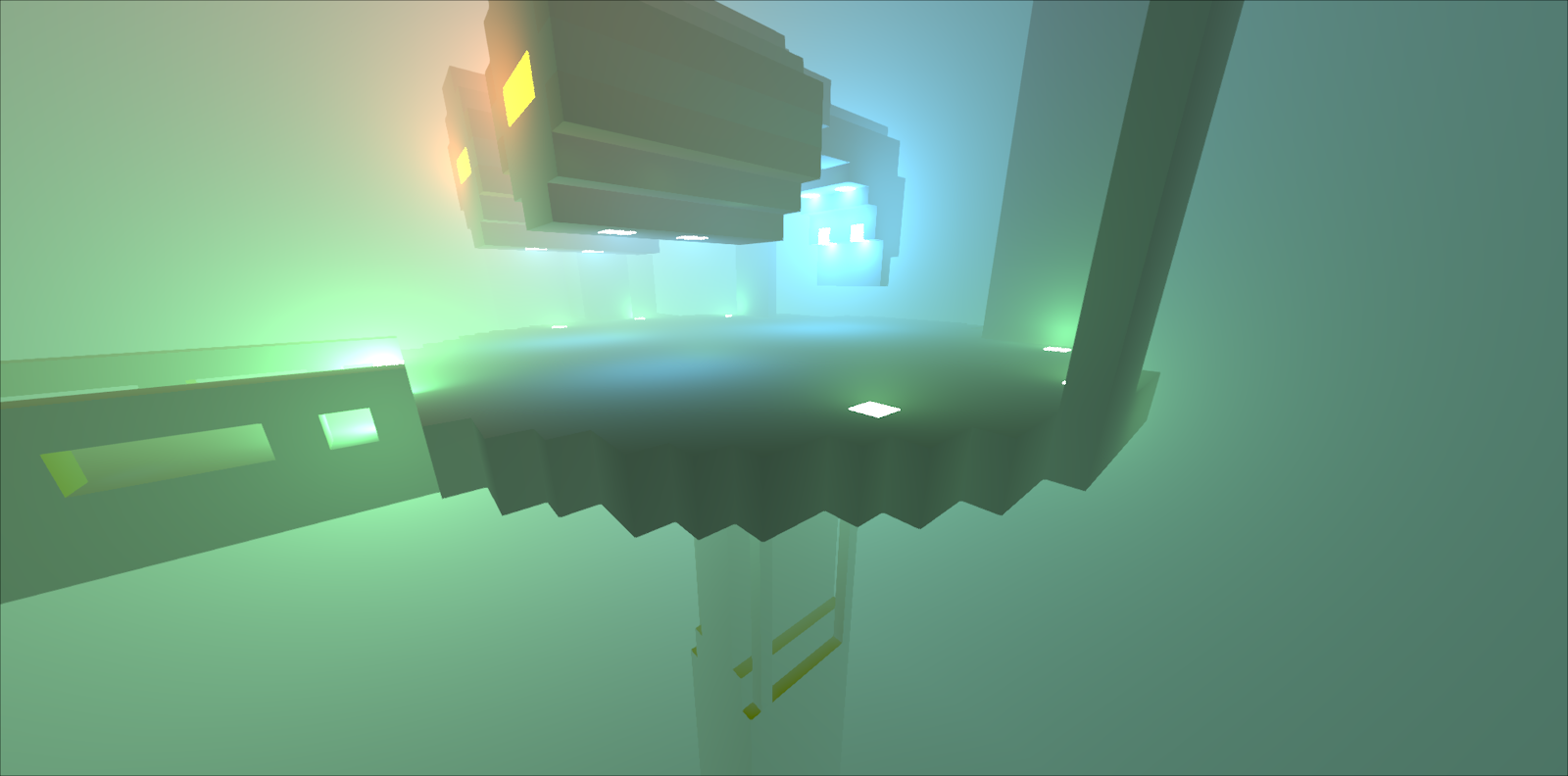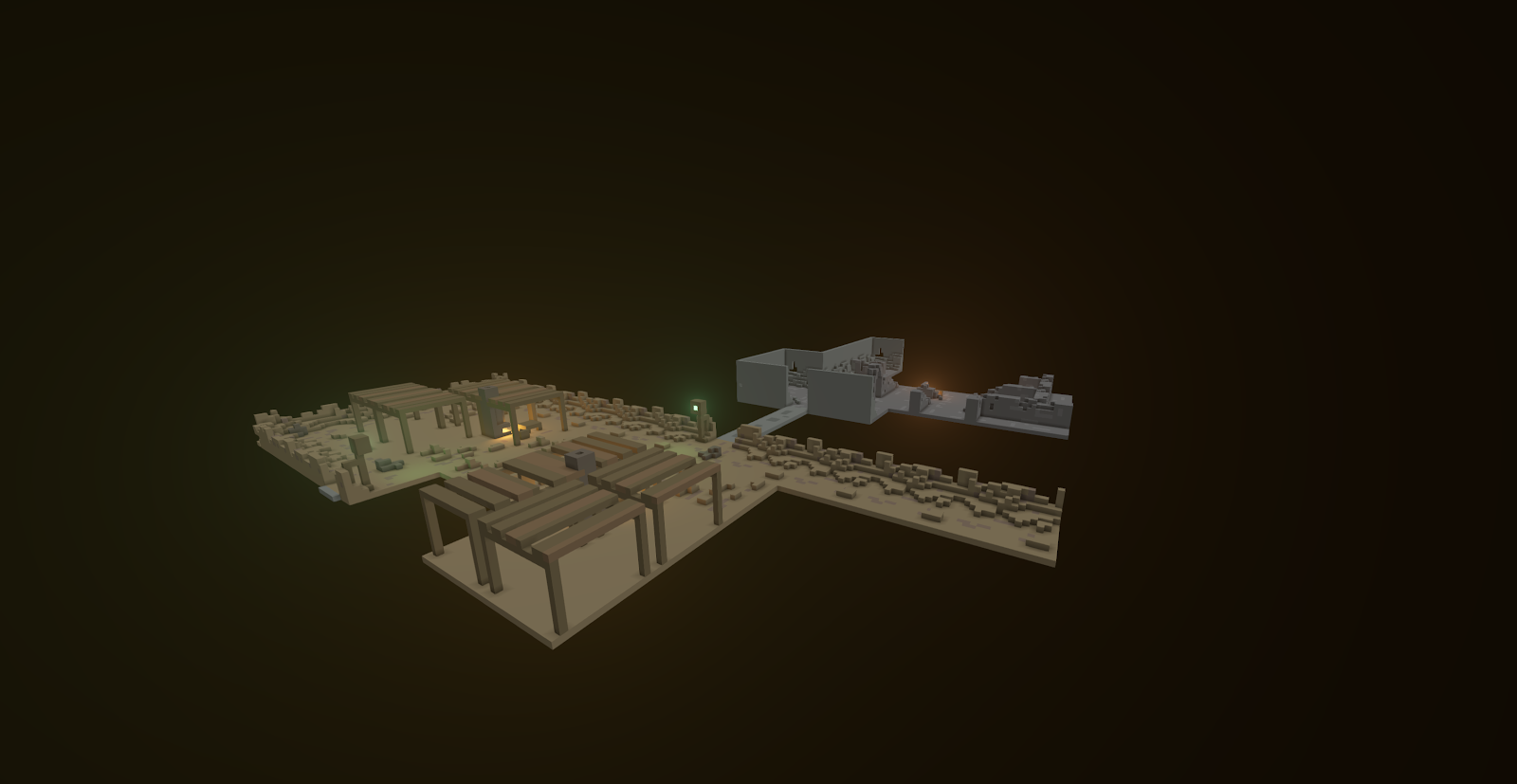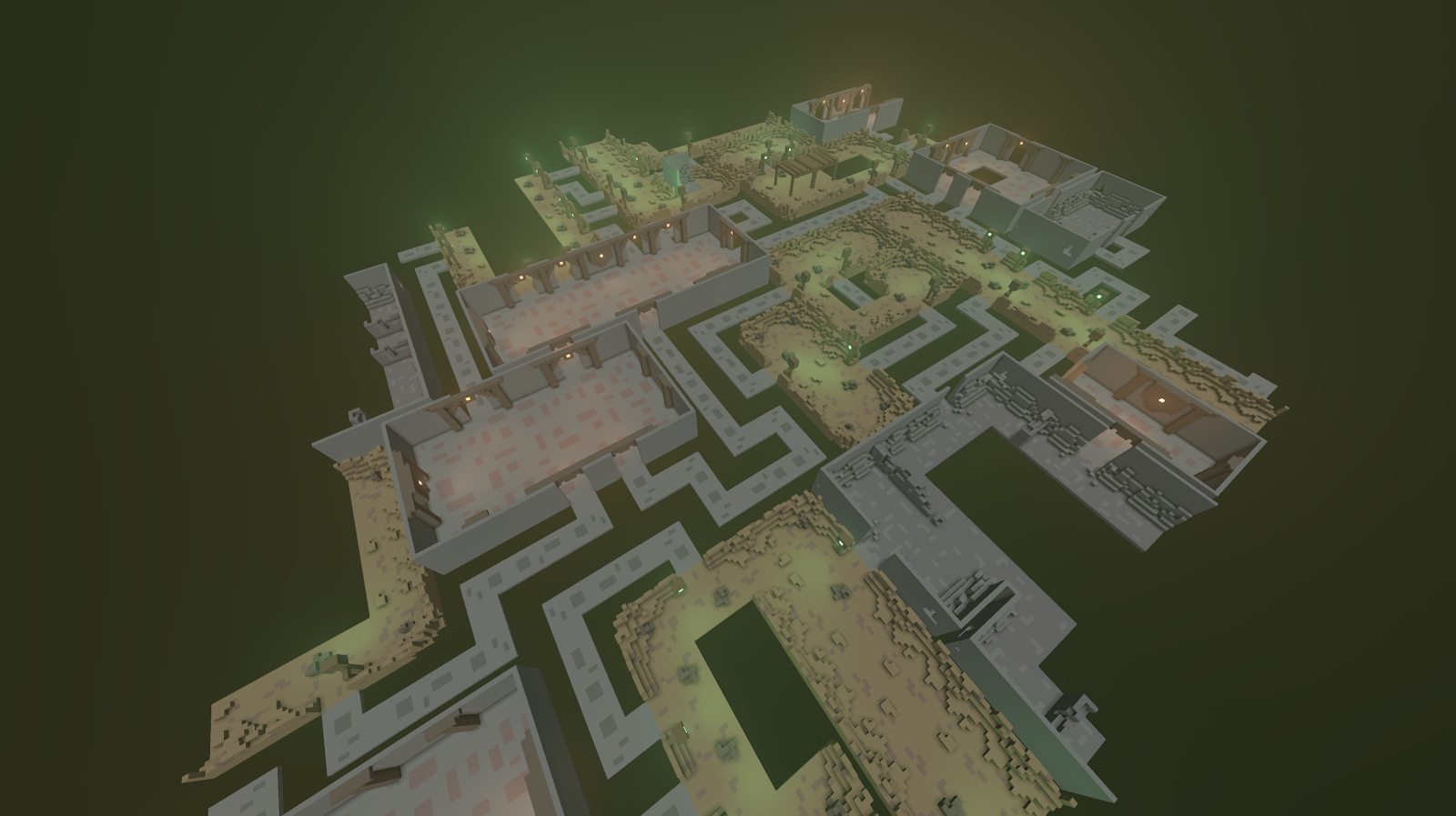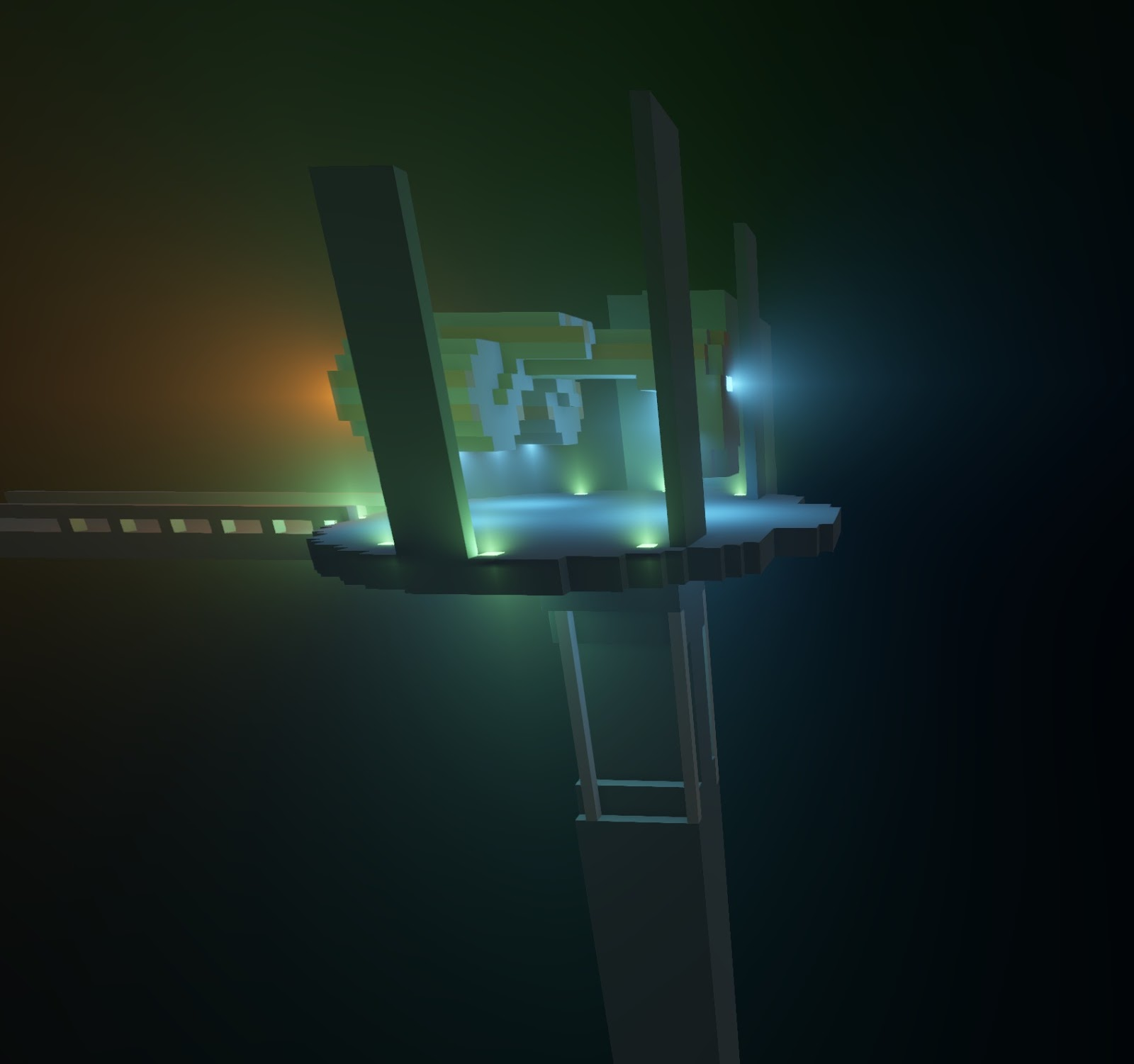I want a simple, fast shader that gives me fog illuminated by point lights. To do this, I created a screen-space effect that achieves the results below. The pipeline is nearly as simple as for plain point lights. It requires no volumetric data structures, no ray marching, and can be tacked on seamlessly to an existing point light shader.

The key insight is that it’s possible to compute in closed form the light that emanates from the fog as it is illuminated by a point light. My approach is to find this formula and plug it into a shader.A little spaceship scene rendered in fog with this technique## Fog Model

The shader makes a few assumptions about the fog we are dealing with. It essentially treats each piece of fog as a tiny, semi transparent, white, diffuse surface.

• The shader assumes that fog scatters light uniformly in all directions. Real fog or smoke does not necessarily have this property, but it’s a fine approximation for the look we’re going for.
• The shader assumes that all wavelengths of light interact with the fog in the same way. In reality, this is often not true. For instance, Rayleigh scattering turns the sky blue by scattering blue light more than other wavelengths.
• The light emanating from the fog varies with the inverse square of the distance from the light source to the fog. This has no basis in reality, but looks fine. Lots of resources exist that explain how fog really scatters light and I’m sure you could extend this method with those formulas.

The results are compelling even with these simplifications in place.

## An Analytic Solution

Given an infinitesimal fog fragment (imagine a little cube of fog), I’ll say that the light being emitted by that fog fragment is

$\text{light} = \frac{1}{(\text{distance from light to fog fragment})^2}$

This is saying that the light coming from each fog fragment falls off with the inverse-square of its distance to the light, just like the light coming from a diffuse surface would.

For neatness, rewrite

$d = \text{distance from light to fog fragment}$ $\text{light} = \frac{1}{d^2}$ $\int_{\text{view line}} \text{light at} \hspace{1ex} x \hspace{1em} dx$

Or more formally:

$L = \text{light position}$ $w = \text{world fragment position}$ $c = \text{camera position}$ $\text{light arriving at camera} = \int_{c}^{w} \frac{1}{|x - L|_2} dx$This integral expresses what we want, but it’s more complicated to evaluate than it needs to be. Since all the variables in the image above are 3-vectors, we effectively have 12 variables to deal with. I’ll eliminate most of these by doing a simple reparametrization of the problem.

I’ll define a new space called light space with

• The view line on the x-axis
• The light on the y-axisNow rather than a line integral through 3-space, I just integrate along the x-axis from the camera to the world fragment. Now I need to rewrite the integral. The distance from a point $$x$$ on the x-axis to the light is $$\sqrt{h^2+x^2}$$. So the integral over the view line becomes

$\int \frac{1}{h^2+x^2} dx$

Solving by hand or with your favorite computer algebra system:

$\int \frac{1}{h^2+x^2} dx = \frac{tan^{-1}(\frac{x}{h})}{h}$

So to find the light coming from the fog for a given view line, I evaluate this integral from the camera to the world fragment. If the camera is at $$x=a$$ and the world fragment is at $$x=b$$, the light arriving at a pixel from the illuminated fog along its view ray is

$\int_a^b \frac{1}{h^2+x^2} dx = \frac{tan^{-1}(\frac{b}{h})}{h} - \frac{tan^{-1}(\frac{a}{h})}{h}$

## Thoughts on Implementation

I was able to implement this shader in my deferred shading pipeline without making any serious modifications. It involved adding around a dozen lines of GLSL code. If you are computing diffuse+specular lighting with point lights already, your point light shader should already have access to camera position, light position, and the position of the world at the current pixel, and that’s all you need!

Handling multiple lights is straightforward. You simply sum the contributions of light scattering from fog for each light. To make the system efficient for many lights, you should be sure to only compute the integral for pixels close enough to the light to have a chance at contributing a visible amount of light to the scene. For instance, in a deferred shading pipeline you can render a spherical mesh around the light so that the shader computing the light contribution only runs for pixels near the light.

I found that the hardest part of implementing this shader is correctly putting your camera and world fragment into light space so that you can take advantage of the simple integral.

## ResultsA small level built with the Generate Worlds algorithm with subtle fog lightingMore lights create a stronger effectBy also tracking information about the direction that the light is pointing, you can create nice shaped lights in the fog